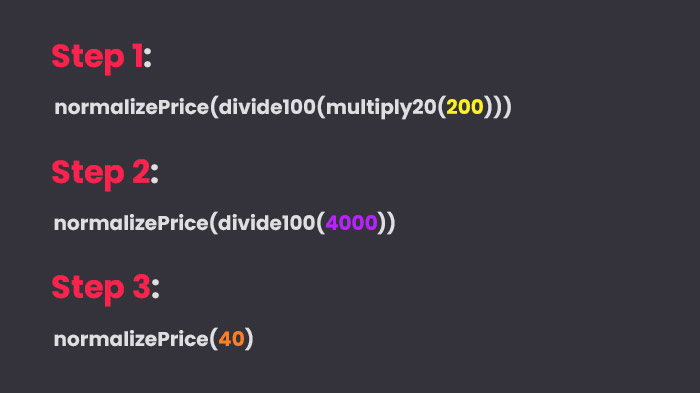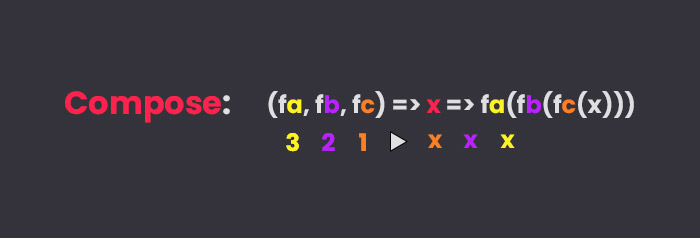# Write better JavaScript, function composition with pipe and compose

10 Nov 2021When I started my journey into functional programming I found myself thinking of it as both exciting and confusing. One of the core concepts I found useful in my everyday work is function composition, thanks to its intuitive nature it’s not hard to introduce it even in a well-established codebase.

## Compose

When speaking of function composition we can think of it as a way of chaining multiple functions together to create a new function, in other terms we are solving a problem reducing it into smaller solutions that in themselves don’t accomplish much but together can solve complex tasks.

Let’s take a look at an example, let’s say we want to calculate a 20% discount on a price, we can create three functions like so:

``````1const multiply20 = (price) => price * 20
2const divide100 = (price) => price / 100
3const normalizePrice = (price) => price.toFixed(2)``````

The code above is really simple, now we need to find a way to combine them, the more traditional way would be to put functions as arguments of the next function:``````1// result = a(b(c(x)))
2const discount = normalizePrice(divide100(multiply20(200))) // 40.00``````

This way the result of the inner function is taken by the outer function as an argument until the end of the chain: We have now managed to chain our functions together, we can achieve the same result writing a compose function improving readability:

``1const compose = (a, b, c) => (x) => a(b(c(x)))``so our code becomes:

``````1const discount = compose(normalizePrice, divide100, multiply20)
2discount(200.0) //40.00``````

Our compose function makes the code more readable and cleaner but we can improve it to accept more than three functions using the higher-order reduceRight function:

``````1const compose =
2  (...fns) =>
3  (x) =>
4    fns.reduceRight((res, fn) => fn(res), x)``````

what we are doing is that using the spread operator we are transforming the arguments (our functions) into an array and return a new function that takes an argument “X” that will be used as the initial value of the accumulator of our reduceRight function. We are basically executing every function passed as an argument from right to left with the result of the previous one. So if we now want to add a new function to add a ‘\$’ prefix to our discount we can simply add it to the compose arguments list

``````1const addPrefix = (price) => "\$" + String(price) //\$ 40.00
2const discountWithPrefix = compose(
4  normalizePrice,
5  divide100,
6  multiply20
7)
8discountWithPrefix(200.0) // '\$40.00'``````

## PipePipe works the exact same way as compose, the only difference is that instead of executing arguments from right to left, it executes them from left to right, we can implement a Pipe function like this:

``````1const pipe =
2  (...fns) =>
3  (x) =>
4    fns.reduce((res, fn) => fn(res), x)``````

the only difference is that reduceRight has become reduce. Personally, I prefer to compose over pipe even if it seems counterintuitive at first glance. Our final code would be:

``````1const multiply20 = (price) => price * 20
2const divide100 = (price) => price / 100
3const normalizePrice = (price) => price.toFixed(2)
4const addPrefix = (price) => "\$" + String(price)
5
6const pipe =
7  (...fns) =>
8  (x) =>
9    fns.reduce((res, fn) => fn(res), x)
10const compose =
11  (...fns) =>
12  (x) =>
13    fns.reduceRight((res, fn) => fn(res), x)
14
15const discountPipe = pipe(multiply20, divide100, normalizePrice, addPrefix)
16const discountCompose = compose(
18  normalizePrice,
19  divide100,
20  multiply20
21)
22
23discountPipe(200) // '\$40.00'
24discountCompose(200) // '\$40.00'``````

## Ramda

You don’t have to write your own version of pipe or compose every time you write a piece of code, you can use libraries such as Ramda and focus on the implementation of your code.